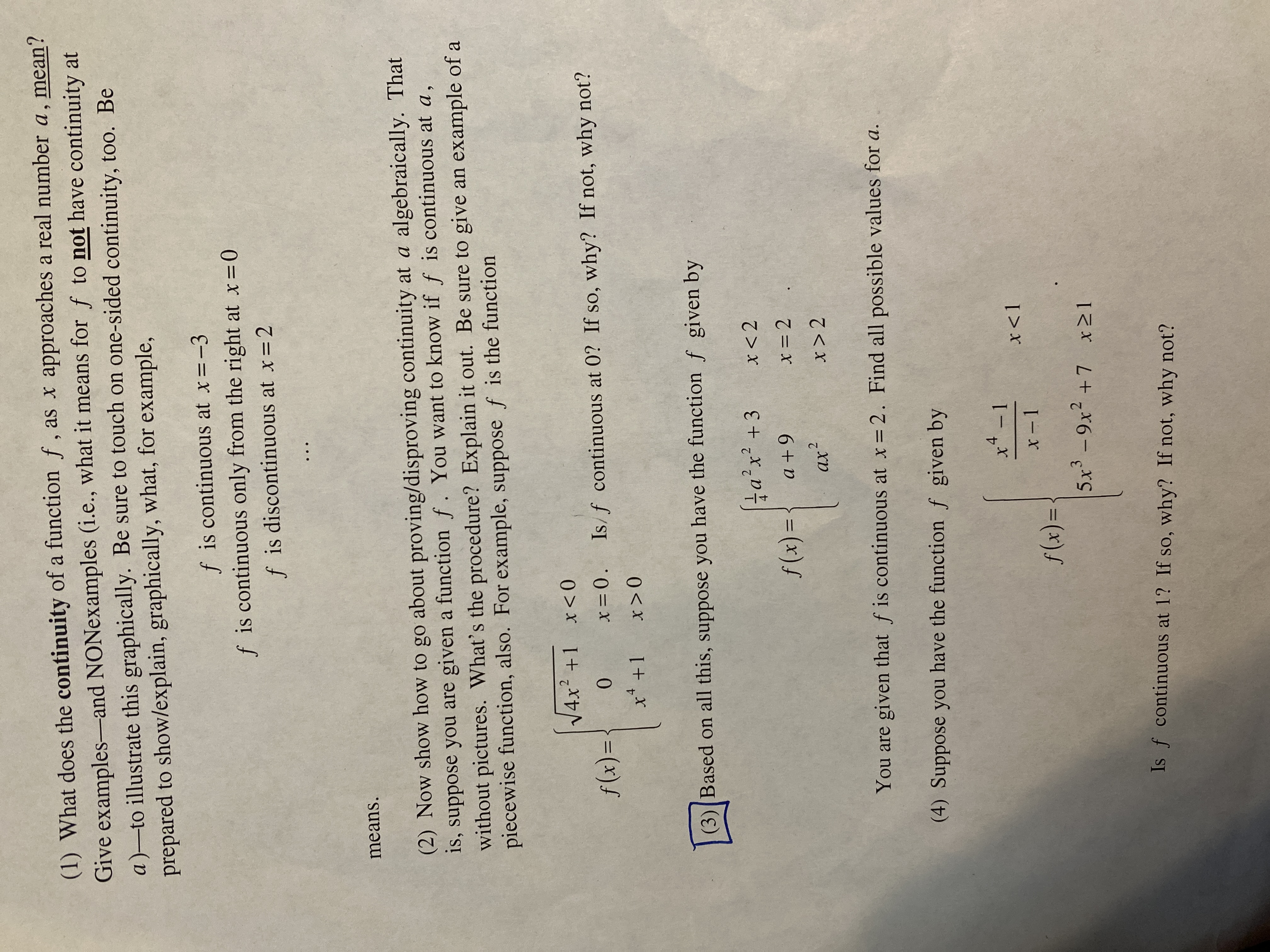# (1) What does the continuity of a function f , as x approaches a real number a, mean? Give examples-and NONexamples (i.e., what it means for f to not have continuity at a)-to illustrate this graphically. Be sure to touch on one-sided continuity, to0. Be prepared to show/explain, graphically, what, for example, f is continuous at x=-3 f is continuous only from the right at x=0 f is discontinuous at x=2 means. (2) Now show how to go about proving/disproving continuity at a algebraically. That is, suppose you are given a function f. You want to know if f is continuous at a, without pictures. What's the procedure? Explain it out. Be sure to give an example of a piecewise function, also. For example, suppose f is the function V4x² +1 x<0 f (x)= 0. x=0. Is/f continuous at 0? If so, why? If not, why not? x* +1 x>0 (3)(Based on all this, suppose you have the function f given by ta'x? +3 f(x) = { a+9 x = 2 .2 ax? x > 2 You are given that f is continuous at x=2. Find all possible values for a. (4) Suppose you have the function f given by 4 x* - 1 x<1 f(x)= 5x³ - 9x2 +7 x21 3 Is f continuous at 1? If so, why? If not, why not?

Question

#3help_outlineImage Transcriptionclose(1) What does the continuity of a function f , as x approaches a real number a, mean? Give examples-and NONexamples (i.e., what it means for f to not have continuity at a)-to illustrate this graphically. Be sure to touch on one-sided continuity, to0. Be prepared to show/explain, graphically, what, for example, f is continuous at x=-3 f is continuous only from the right at x=0 f is discontinuous at x=2 means. (2) Now show how to go about proving/disproving continuity at a algebraically. That is, suppose you are given a function f. You want to know if f is continuous at a, without pictures. What's the procedure? Explain it out. Be sure to give an example of a piecewise function, also. For example, suppose f is the function V4x² +1 x<0 f (x)= 0. x=0. Is/f continuous at 0? If so, why? If not, why not? x* +1 x>0 (3)(Based on all this, suppose you have the function f given by ta'x? +3 f(x) = { a+9 x = 2 .2 ax? x > 2 You are given that f is continuous at x=2. Find all possible values for a. (4) Suppose you have the function f given by 4 x* - 1 x<1 f(x)= 5x³ - 9x2 +7 x21 3 Is f continuous at 1? If so, why? If not, why not? fullscreen

### Want to see this answer and more?

Experts are waiting 24/7 to provide step-by-step solutions in as fast as 30 minutes!*

*Response times vary by subject and question complexity. Median response time is 34 minutes and may be longer for new subjects.
Tagged in
MathCalculus

### Limits